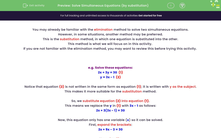# Solve Simultaneous Equations (by substitution)

In this worksheet, students will learn how two solve linear simultaneous equations using the substitution method.Key stage:  KS 4

Year:  GCSE

GCSE Subjects:   Maths

GCSE Boards:   Pearson Edexcel, OCR, Eduqas, AQA,

Curriculum topic:   Algebra

Curriculum subtopic:   Solving Equations and Inequalities Algebraic Equations

Difficulty level:#### Worksheet Overview

You may already be familiar with the elimination method to solve two simultaneous equations.

However, in some situations, another method may be preferred.

This is the substitution method, in which one equation is substituted into the other.

This method is what we will focus on in this activity.

If you are not familiar with the elimination method, you may want to review this before trying this activity.

e.g. Solve these equations:

2x + 3y = 30  (1)

y = 3x - 1  (2)

Notice that equation (2) is not written in the same form as equation (1); it is written with y as the subject.

This makes it more suitable for the substitution method.

So, we substitute equation (2) into equation (1).

This means we replace the y in (1) with 3x - 1 as follows:

2x + 3(3x - 1) = 30

Now, this equation only has one variable (x) so it can be solved.

First, expand the brackets:

2x + 9x - 3 = 30

Now, simplify:

11x - 3 = 30

Now, solve:

11x = 33

x = 3

Now, let's substitute x = 3 into either (1) or (2), whichever you think is easiest.

Here, we have substituted into (2):

y = 3 x 3 - 1

y = 8

As before we can check our solutions using equation (1):

2 x 3 + 3 x 8 = 30

6 + 24 = 30 [correct]

So x = 3 and y = 8.

The substitution method is to be preferred over the elimination method when one equation is written in the form y = ... or x = ... and the other is in the form ax + by = c where a, b, c are constants.

Now its time for you to try some!

In this activity, we will solve pairs of simultaneous equation by substituting one equation into the other, then simplifying and solving from there.

We can only do this when one equation is in the form y = ... or x = ... and the other is in the form b.

Make sure you have a pen and paper handy to record your working.

### What is EdPlace?

We're your National Curriculum aligned online education content provider helping each child succeed in English, maths and science from year 1 to GCSE. With an EdPlace account you’ll be able to track and measure progress, helping each child achieve their best. We build confidence and attainment by personalising each child’s learning at a level that suits them.

Get started